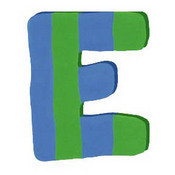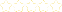• > Crystal Kirch
• > Unit E - Basics of Polynomials
+# Unit E - Basics of Polynomials

Owner: Crystal Kirch
##### Description:
This unit focuses on characteristics of polynomials. A polynomial is defined as an function with real-number coefficients and non-negative, whole-number exponents. Its graph is continuous (don’t have to lift your pencil from the paper to draw it) and has smooth, rounded turns (no sharp points like an absolute value graph) A polynomial can have one term (monomial), two terms (binomial), three terms (trinomial), or four+ terms (polynomial). In addition, a polynomial is described by the largest exponent that it has in the equation. If the largest exponent is 0, it is a constant function. If the largest exponent is 1, it is linear; if it is 2, it is quadratic; if it is 3, it is cubic; if it is 4, it is quartic; if it is 5, it is quintic; if it is anything 6 or larger, it is just “degree 6” or “degree 7” (and so on). We will begin by looking at quadratic functions in more detail. We are familiar with the name parabola to describe the graphs of quadratics. However, we will be examining those graphs and identifying key parts, such as the vertex (which is either a maximum or a minimum), intercepts, and axis. Next, we will begin our journey through the world of general polynomials, focus on cubics, quartics, and quintics. We will look at their end behavior, their zeroes, their intercepts, and other characteristics that define these graphs, including a review of extrema and intervals of increase and decrease.

### Your Quiz Points in this Playlist

Total Possible
0
7 Tutorials in this Playlist

#### Unit E Concept 1:Identifying all parts of quadratics and graphing them.

Author: Crystal Kirch
Rating:(2)

#### Unit E Concept 2: Quadratic Applications (Path of Football)

Author: Crystal Kirch
Rating:(1)

#### Unit E Concept 3: Quadratic Applications (Max Area)

Author: Crystal Kirch
Rating:(3)

#### Unit E Concept 4: Using the leading coefficient test for polynomials to write end behavior

Author: Crystal Kirch
Rating:(1)

#### Unit E Concept 5-6: Finding zeroes/mult using factoring & Writing eq. of poly given zeroes/mult

Author: Crystal Kirch
Rating:(2)

#### Unit E Concept 7a: Graphing polynomials, identifying all parts

Author: Crystal Kirch
Rating:(1)

#### Unit E Concept 7b: Graphing polynomials, identifying all parts

Author: Crystal Kirch
Rating:(0)